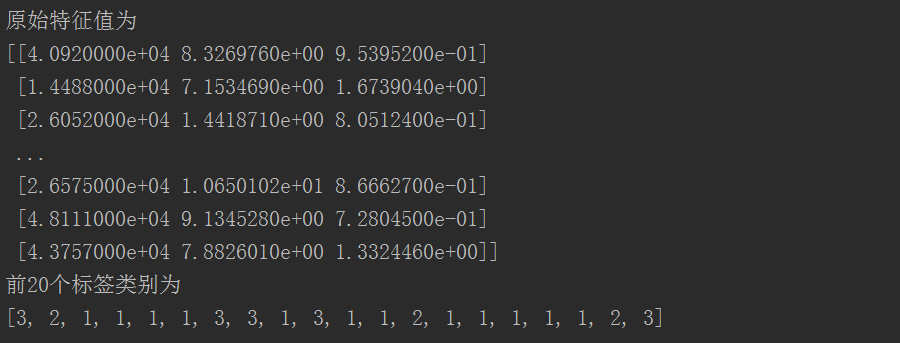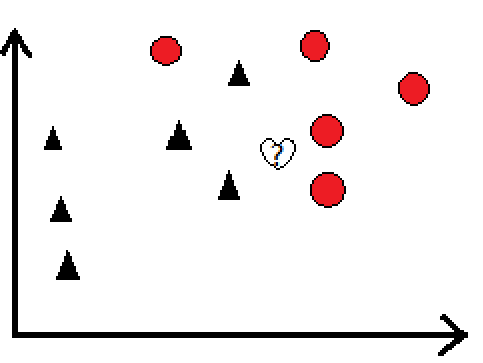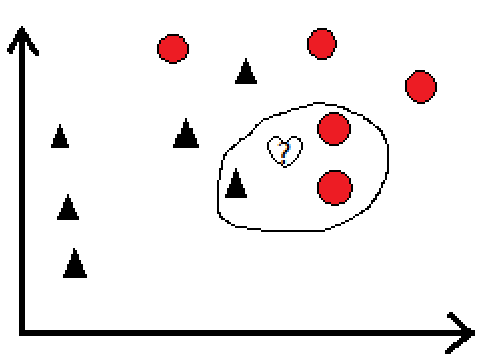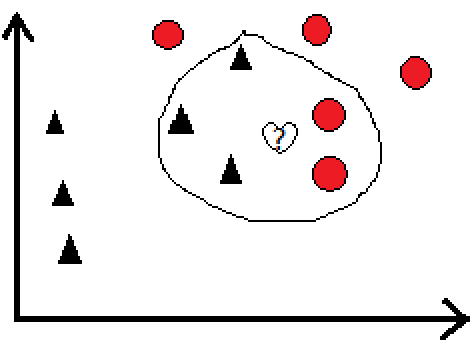2018-12-14 12:59:36 Sophia_11 阅读数 71
• ###### 10小时玩转机器学习

购买课程后，可扫码进入学习群，获取唐宇迪老师答疑 课程主要包括人工智能五大核心模块：人工智能改数与K近邻算法实战，线性回归算法，经典分类算法-逻辑回归，决策树算法，贝叶斯算法，无监督算法-聚类与PCA降维。风格通俗易懂，唐宇迪老师将用接地气的方式讲解复杂算法问题，以实战为导向，基于真实数据集从零开始，带领大家一步步完成整个人工智能项目。

2189 人正在学习 去看看 唐宇迪

# 本文是《机器学习从零到掌握》系列之第3篇

（1）每年获得飞行常客里程数

（2）玩视频游戏所耗时间百分比

（3）每周消费的冰淇淋公升数

file2matrix.py

#!/usr/bin/env python
# -*- coding:utf-8 -*-
from numpy import *
import operator
import matplotlib.pyplot as plt

def file2matrix(filename):
"""将文本记录转换为Numpy的解析程序"""
fr = open(filename)
number_lines = len(array_lines)  # 获取文件行数
return_mat = zeros((number_lines, 3))  # 创建返回的Numpy矩阵，大小为number_lines*3
class_label_vector = []     # 类别标签列表
index = 0
for line in array_lines:
line = line.strip()  # 截取掉所有的回车符
list_from_line = line.split('\t')  # 使用tab字符\t将上一步得到的整行数据分割成一个元素列表
return_mat[index, :] = list_from_line[0:3]  # 取前3个元素，将它们存储到特征矩阵中
# 将列表的最后一列存储在类别便签列表内，必须明确表示存储的是整型，不然默认当成字符串处理
class_label_vector.append(list_from_line[-1])   # 将列表的最后一列存储在类别便签列表内
index += 1
return return_mat, class_label_vector

# 创建一个读取数据实例，并输出数据
dating_data_mat, dating_labels = file2matrix('datingTestSet2.txt')
print("原始特征值为")
print(dating_data_mat)
print("前20个标签类别为")
print(dating_labels[:20])k近邻算法 机器学习 相关内容

2018-11-08 17:09:35 qq_41682922 阅读数 122
• ###### 10小时玩转机器学习

购买课程后，可扫码进入学习群，获取唐宇迪老师答疑 课程主要包括人工智能五大核心模块：人工智能改数与K近邻算法实战，线性回归算法，经典分类算法-逻辑回归，决策树算法，贝叶斯算法，无监督算法-聚类与PCA降维。风格通俗易懂，唐宇迪老师将用接地气的方式讲解复杂算法问题，以实战为导向，基于真实数据集从零开始，带领大家一步步完成整个人工智能项目。

2189 人正在学习 去看看 唐宇迪

**

## 机器学习之入门级算法K近邻

**

K近邻算法与逻辑回归不同的是: K近邻可以解决多分类问题，而逻辑回归是解决二分类问题的（但是工作当中逻辑回归用的还是比较多的）• 计算测试数据与各个训练数据之间的距离
• 按照距离的递增关系进行排序
• 选取距离最小的k个点
• 确定前k个点所在类别的出现频率
• 返回前k个点钟出现频率最高的类别作为测试数据的预测分类

							  **原创**


k近邻算法 机器学习 相关内容

2017-09-10 20:35:23 justry24 阅读数 591
• ###### 10小时玩转机器学习

购买课程后，可扫码进入学习群，获取唐宇迪老师答疑 课程主要包括人工智能五大核心模块：人工智能改数与K近邻算法实战，线性回归算法，经典分类算法-逻辑回归，决策树算法，贝叶斯算法，无监督算法-聚类与PCA降维。风格通俗易懂，唐宇迪老师将用接地气的方式讲解复杂算法问题，以实战为导向，基于真实数据集从零开始，带领大家一步步完成整个人工智能项目。

2189 人正在学习 去看看 唐宇迪

KNN算法没有显式的学习过程，事实上，它是“懒惰学习”的代表：在训练阶段仅仅是把样本保存起来，训练时间开销为0

（平均法）：
import numpy as np

def knnclassify(inX, dataSet, labels, k):
dataSetSize = dataSet.shape
diffMat = np.tile(inX, (dataSetSize,1)) - dataSet
sqDiffMat = diffMat**2
sqDistances = sqDiffMat.sum(axis=1)
distances = sqDistances**0.5
sortedDistIndicies = distances.argsort()
classCount = {}
for i in range(k):
voteIlabel = labels[sortedDistIndicies[i]]
classCount[voteIlabel] = classCount.get(voteIlabel, 0) + 1
sortedClassCount = sorted(classCount.items(), key=lambda classCount : classCount, reverse=True)
return sortedClassCount

（加权投票法）

classCount[votelabel] = classCount.get(votelabel, 0) + round((1.5 - i / (k-1)), 2)

 k 错误总数（平均投票） 错误总数（加权投票） 1 13 13 2 13 13 3 10 13 4 11 10 5 17 11 6 17 14 7 21 18 8 18 16 9 21 19

1. 并不是 K 越大，分类准确率越高。
2. 当 K 越大时，加权投票的分类 优势越明显

import numpy as np
from knn.KNNclassifier import knnclassify
# 导入当前文件夹下的文件，(如果不加入"knn.",则会出现红色波浪线，但可正常运行）
def creatrDateSet():
group = np.array([[1.0,1.1],[1.0,1.0],[0,0],[0,0.1]])
labels = ['A','A','B','B']
return group, labels

group, labels = creatrDateSet()
print(knnclassify([0, 0], group, labels, 3))  # 结果B
print(knnclassify([1, 1], group, labels, 4))  # 结果A
print(knnclassify([0.5, 0.5], group, labels, 4))  # 结果B

def classify0(inX, dataSet, labels, k):
dataSetSize = dataSet.shape #4
diffMat = np.tile(inX, (dataSetSize,1)) - dataSet
#  np.tile(inX, (dataSetSize,1) 结果为 [[0,0],[0,0],[0,0],[0,0]]
# 减去dataSet 后每行为每个点的距离差
sqDiffMat = diffMat**2
# 将每个距离差平方
sqDistances = sqDiffMat.sum(axis=1)
# #axis=0表述列 ，axis=1表述行, 横项相加
distances = sqDistances**0.5

# 开根号得到距离:[ 1.48660687  1.41421356  0.          0.1      ]
sortedDistIndicies = distances.argsort() # 返回结果从头到尾为非降序排序的index：[2 3 1 0]

classCount={}
for i in range(k):
voteIlabel = labels[sortedDistIndicies[i]]
# 循环取出排序从近到远的labels  : B B A
classCount[voteIlabel] = classCount.get(voteIlabel,0) + 1 # 构建字典，最终得到频次{'B': 2, 'A': 1}

sortedClassCount = sorted(classCount.items(), key= lambda classCount:classCount, reverse=True)
# operator模块提供的itemgetter函数用于获取对象的哪些维的数据
# sortedClassCount = sorted(classCount.iteritems(), key=operator.itemgetter(1), reverse=True)
'''在Python2.x中，items( )用于 返回一个字典的拷贝列表【Returns a copy of the list of all items (key/value pairs) in D】，占额外的内存。
iteritems() 用于返回本身字典列表操作后的迭代【Returns an iterator on all items(key/value pairs) in D】，不占用额外的内存。
Python 3.x 里面，iteritems() 和 viewitems() 这两个方法都已经废除了，而 items() 得到的结果是和 2.x 里面 viewitems() 一致的。
在3.x 里 用 items()替换iteritems() ，以列表返回可遍历的(键, 值) 元组数组'''

print(sortedClassCount)
return sortedClassCount
# sortedClassCount 得到的值为列表，列表元素为每个标签和频次，由频次从高到底排序 ，本例中为[('B', 2), ('A', 1)]

group, labels = creatrDateSet()
print(classify0([0, 0], group, labels, 3))  # 结果B
print(classify0([1, 1], group, labels, 4))  # 结果A
print(classify0([0.5, 0.5], group, labels, 4))  # 结果B

import numpy as np
from knn.KNNclassifier import knnclassify

# 将 文本记录 转换为 NumPy
def file2matrix(filename, dim):
fr = open(filename)
numberOfLines = len(fr.readlines())        #get the number of lines in the file
returnMat = np.zeros((numberOfLines,dim))        #prepare matrix to return
classLabelVector = []                      #prepare labels return
fr = open(filename)
index = 0
line = line.strip()
listFromLine = line.split('\t')
returnMat[index,:] = listFromLine[0:dim]
classLabelVector.append(int(listFromLine[-1]))
index += 1
return returnMat,classLabelVector

def normalize(dataSet):
minVals = dataSet.min(0) # 每列最小值
maxVals = dataSet.max(0)
ranges = maxVals - minVals
normDataSet = np.zeros(np.shape(dataSet))
m = dataSet.shape
normDataSet = dataSet - np.tile(minVals, (m, 1))
normDataSet = normDataSet/np.tile(ranges, (m, 1))
return normDataSet , ranges, minVals

def dataplot(dataSet, labels):
import  matplotlib.pyplot as plt
fig = plt.figure()
ax.scatter(dataSet[:,1], dataSet[:,2], 15.0*np.array(labels), np.array(labels))
ax.set_ylabel('Ice Cream Consumption')
ax.set_xlabel('Time of Playing Games')
# 后面两个参数分别控制散点大小和颜色
plt.show()

def classifytest(dataset, datalabel, k, testRatio):
m = dataset.shape # 数据列数
numTestVecs = int(m * testRatio) #此次测试所用的样本数目
errorCount = 0.0
for i in range(numTestVecs):
classifierResult = knnclassify(dataset[i, :], dataset[numTestVecs:m, :], datalabel[numTestVecs:m], k)
#print("the classifier came back with: %d, the real answer is: %d" % (classifierResult, datalabel[i]))
# dataset 前 测试率*数据总数目 用于测试，  使用 余下的作为分类依据
if (classifierResult != datalabel[i]):
errorCount += 1.0
print("the total error rate is: %f" % (errorCount / float(numTestVecs)))

def main():

datingDataMat, datingLabels = file2matrix('datingTestSet2.txt',3)
normMat, ranges, minVals = normalize(datingDataMat)
dataplot(normMat, datingLabels)
#classifytest(normMat,datingLabels,3,0.1) # 第三个参数为分类器的k值
# 第四个系数为测试样本比例，系数越高 分类准确率越低（用于测试的越多，用于分类的越少）

resultList = ['not at all', 'in small dose', 'in large doses']
timeofgame = float(input("percentage of time spend playing video games:"))
flymiles = float(input("frequent flier miles earner per year:"))
icecream = float(input("liters of ice cream consumed per year:"))
inarr = np.array([timeofgame, flymiles, icecream])
norminput = (inarr - minVals) / ranges
result = knnclassify(norminput, normMat, datingLabels, 3)
print("you will probable like this person", resultList[result - 1])

if __name__=="__main__":
main()

import numpy as np
from os import listdir
from knn.KNNclassifier import knnclassify

# 将 32*32 的数组，转化为一维 nd.array 矩阵
def img2vector(filename):
returnVect = np.zeros((1,1024))
fr = open(filename)
for i in range(32):
for j in range(32):
returnVect[0,32*i+j] = int(lineStr[j])
return returnVect

def handwritingClassTest():
hwLabels = []
trainingFileList = listdir('digits/trainingDigits')          #load the training set
m = len(trainingFileList)  # 文件数目，同时也是训练样本数目 本例中为1934
trainingMat = np.zeros((m,1024))
for i in range(m):
fileNameStr = trainingFileList[i]
fileStr = fileNameStr.split('.')    #take off .txt
classNumStr = int(fileStr.split('_'))
hwLabels.append(classNumStr)  # 得到训练样本的真实标签
trainingMat[i,:] = img2vector('digits/trainingDigits/%s' % fileNameStr)
# 对每个文件进行 向量化处理，得到 m * 1024 的矩阵

testFileList = listdir('digits/testDigits')        #iterate through the test set
errorCount = 0.0
mTest = len(testFileList)
for i in range(mTest):
fileNameStr = testFileList[i]
fileStr = fileNameStr.split('.')    # 去掉 .txt 的后缀
classNumStr = int(fileStr.split('_'))  # _ 前为该数字的正确值
vectorUnderTest = img2vector('digits/testDigits/%s' % fileNameStr)
classifierResult = knnclassify(vectorUnderTest, trainingMat, hwLabels, 3)  #对每个测试样本进行分类
#print("the classifier came back with: %d, the real answer is: %d" % (classifierResult, classNumStr))
if (classifierResult != classNumStr): errorCount += 1.0
print("\nthe total number of errors is: %d" % errorCount)
print("\nthe total error rate is: %f" % (errorCount/float(mTest)))

handwritingClassTest()
# 约会选择中，将一份数据一分为2位训练集和测试集，而此例中为分开好的
# 由于数据只能为 0 和 1， 因此无需归一化处理

k近邻算法 机器学习 相关内容

2017-10-04 14:44:29 qq_33267669 阅读数 334
• ###### 10小时玩转机器学习

购买课程后，可扫码进入学习群，获取唐宇迪老师答疑 课程主要包括人工智能五大核心模块：人工智能改数与K近邻算法实战，线性回归算法，经典分类算法-逻辑回归，决策树算法，贝叶斯算法，无监督算法-聚类与PCA降维。风格通俗易懂，唐宇迪老师将用接地气的方式讲解复杂算法问题，以实战为导向，基于真实数据集从零开始，带领大家一步步完成整个人工智能项目。

2189 人正在学习 去看看 唐宇迪

## 概述

k近邻法不具有显式的学习过程，实际上是利用训练数据集对特征向量空间进行划分，并作为其分类的模型。
k值的选择, 距离度量分类决策规则是k近邻算法的三个基本要素。### 距离度量

Lp$L_p$距离的定义如下：

Lp(xi,xj)=[l=1nxlixljp]1pL2(xi,xj)=[l=1nxlixlj2]12

L1(xi,xj)=l=1nxlixlj

## k近邻算法

1. 根据给定的距离度量，在训练集T中找出与x最近邻的k个点，涵盖着k个点的x的邻域记作Nk(x)$N_k(x)$
2. Nk(x)$N_k(x)$中根据分类决策规则决定x的类别y

## 实现k近邻算法

### 线性扫描法

#### 数据导入

import numpy as np
from operator import itemgetter

def get_train_data(filename):
return np.array([label.strip().split() for label in open(filename)])

# 将数据以字符串类型读入numpy数组
train_data = get_train_data('datingTestSet.txt')
# 提取训练数据及对应的类别
train_data, labels = train_data[:,:3].astype(float), train_data[:,3]
# 将类别转化为数值类型,每个数值代表一个类别
index_labels = list(set(labels))
labels = [index_labels.index(label) for label in labels.tolist()]

#### 特征值归一化

array([['40920', '8.326976', '0.953952', 'largeDoses'],
['14488', '7.153469', '1.673904', 'smallDoses'],
['26052', '1.441871', '0.805124', 'didntLike'],
...,
['26575', '10.650102', '0.866627', 'largeDoses'],
['48111', '9.134528', '0.728045', 'largeDoses'],
['43757', '7.882601', '1.332446', 'largeDoses']],
dtype='|S10')

(4092014488)2+(8.3269767.153469)2+(0.9539521.673904)2

new_value = (old_value - min) / (max - min)

def autoNorm(dataSet):
min_value = dataSet.min(0) # 0代表axis=0，获取每列最小值
max_value = dataSet.max(0)
ranges = max_value - min_value
m = dataSet.shape
norm_dataSet = dataSet - np.tile(min_value, (m, 1)) # np.tile()复制第一个参数为第二个参数给出的形状
norm_dataSet /= np.tile(ranges, (m, 1)) # numpy的/代表矩阵对应元素相除
return norm_dataSet, ranges, min_value

norm_mat, ranges, min_value = autoNorm(train_data)

array([[ 0.44832535,  0.39805139,  0.56233353],
[ 0.15873259,  0.34195467,  0.98724416],
[ 0.28542943,  0.06892523,  0.47449629],
...,
[ 0.29115949,  0.50910294,  0.51079493],
[ 0.52711097,  0.43665451,  0.4290048 ],
[ 0.47940793,  0.3768091 ,  0.78571804]])

#### 分类

# inX: 被分类的输入向量
# labels：数据集中每个样本的分类，和dataSet对应
# k: 选择最近邻个数
def classify0(inX, dataSet, labels, k):
# 计算当前输入向量与训练集中所有点之间的距离， 并按距离依次排序
diff_mat = np.tile(inX, (dataSet.shape, 1)) - dataSet
distances = ((diff_mat**2).sum(axis=1))**0.5
sorted_distances_index = distances.argsort() # 从小到大排序， 返回数组对应值的索引
# 选取k个点
class_count = {}
for i in range(k):
vote_label = labels[sorted_distances_index[i]]
class_count[vote_label] = class_count.get(vote_label, 0) + 1
# 确定前k个点的对应类别出现频率， 并按频率排序
sorted_class_count = sorted(class_count.items(), key=itemgetter(1), reverse=True)
# 返回最近的k个实例中出现次数最多的类别
return sorted_class_count

#### 测试

def datingClassTest(norm_data_set, labels):
# 选取训练集个数的10%作为测试数据，其余90%作为已知样本点
num_test_vecs = int(norm_data_set.shape * 0.1)
error_count = 0
for i in range(num_test_vecs):
classifier_result = classify0(norm_data_set[i,:], norm_data_set[num_test_vecs:, :], labels, 3)
if classifier_result != labels[i]: error_count += 1
return float(error_count) / num_test_vecs

### kd树法

kd树是二叉树，表示对k维空间的一个划分，构造kd树相当于不断地用垂直于坐标轴的超平面将k维空间切分，构成一系列的k维超矩形区域。

kd树就是把整个空间划分为特定的几个部分，然后在特定空间的部分内进行相关搜索操作。通常依次选择坐标轴对空间进行切分，选择训练实例点在选定坐标轴上的中位数为切分点，这样得到的kd树是平衡的，但平衡kd树的搜索效率未必是最优的。

#### 构建kd树class KDNode(object):
def __init__(self, dom_elt, split, left_node, right_node):
self.dom_elt = dom_elt # 保存结点数据
self.split = split # 进行分割的维度
self.left_node = left_node
self.right_node = right_node

class KDTree(object):

def __init__(self, data_set):
# 取数据维度
k = data_set.shape

def create_node(split, data_set):
if data_set.shape == 0: return None
# 按某列排序，并返回排序后原数组的索引
sorted_index = np.argsort(data_set[:,split], axis=0)
new_data_set = data_set[sorted_index]
# 取中位数所在索引
split_index = new_data_set.shape // 2 # //为整除
median_data = new_data_set[split_index]
next_split = (split + 1) % k # 选择切分坐标轴
return KDNode(median_data, split, create_node(next_split, new_data_set[:split_index]), create_node(next_split, new_data_set[split_index + 1:]))

# 确定初始split域
# 计算每个维度的数据的方差
var_result = np.var(data_set, axis=0)
split_num = list(var_result).index(var_result.max())
self.root_node = create_node(split_num, data_set)

#### kd树的最近邻搜索

1. 找出包含目标点x的叶结点：从根结点出发，递归地向下访问，若目标点x当前维的坐标小于被访问结点的切分点的坐标，则移动到左子结点，否则移动到右子结点，直到叶结点，以此叶结点为当前最近点
2. 从叶结点开始递归地向上回退，在每个结点处进行以下操作：
a. 若该实例点比当前最近点与目标点的距离更近，则以该实例点为当前最近点
b. 检查该结点的另一子结点对应的区域是否与以目标点为球心、以目标点与当前最近点之间的距离为半径的超球体相交。若相交，则移动到另一子结点递归进行最近邻搜索；若不相交则向上回退。
3. 回退到根结点时，搜索结束，当前最近点为目标点x的最近邻点。from collections import namedtuple

class KDNode(object):
def __init__(self, dom_elt, split, left_node, right_node):
self.dom_elt = dom_elt # 保存结点数据
self.split = split # 进行分割的维度
self.left_node = left_node
self.right_node = right_node

find_result = namedtuple('Result_tuple', 'nearest_point nearest_dist nodes_visited')

class KDTree(object):

def __init__(self, data_set):
# 取数据维度
k = data_set.shape

def create_node(split, data_set):
if data_set.shape == 0: return None
# 按某列排序，并返回排序后原数组的索引
sorted_index = np.argsort(data_set[:,split], axis=0)
new_data_set = data_set[sorted_index]
# 取中位数所在索引
split_index = new_data_set.shape // 2
median_data = new_data_set[split_index]
next_split = (split + 1) % k
return KDNode(median_data, split, create_node(next_split, new_data_set[:split_index]), create_node(next_split, new_data_set[split_index + 1:]))

# 确定split域
var_result = np.var(data_set, axis=0)
split_num = list(var_result).index(var_result.max())
self.root_node = create_node(split_num, data_set)

# KD树的最近邻搜索 (k=1)
def find(self, target_point):
# 取数据维度 (不同于k近邻的k)
k = len(target_point)

def travel(kd_node, target_point, min_dist):
if kd_node is None: return find_result(*k, float('inf'), 0)
# 找到叶结点
nodes_visited = 1
split = kd_node.split # 进行分割的维度
pivot = kd_node.dom_elt # 当前结点的数据
if target_point[split] <= pivot[split]:
nearer_node = kd_node.left_node
further_node = kd_node.right_node
else:
nearer_node = kd_node.right_node
further_node = kd_node.left_node
# 从叶结点回退，直到根结点
nearest_point, nearest_dist, return_visited1 = travel(nearer_node, target_point, min_dist)
nodes_visited += return_visited1
if nearest_dist < min_dist:
min_dist = nearest_dist
if min_dist < abs(pivot[split] - target_point[split]): # 超球体与超平面是否相交
return find_result(nearest_point, nearest_dist, nodes_visited) # 不相交直接向上回退
# 相交则转到另一子结点
temp_dist = np.sqrt(np.sum((pivot - target_point)**2)) # 计算欧式距离
if temp_dist < nearest_dist:
nearest_point = pivot
nearest_dist = temp_dist
min_dist = temp_dist
# 检查另一个子结点是否为更近的点
nearest_point2, nearest_dist2, return_visited2 =travel(further_node, target_point, min_dist)
nodes_visited += return_visited2
if nearest_dist2 < temp_dist:
nearest_point = nearest_point2
nearest_dist = nearest_dist2
return find_result(nearest_point, nearest_dist, nodes_visited)
return travel(self.root_node, target_point, float('inf'))

kdtree =KDTree(np.array([[2,3], [5,4], [9,6], [4,7], [8,1], [7,2]]))
kdtree.find(np.array([3, 4.5]))

Result_tuple(nearest_point=array([2, 3]), nearst_dist=1.8027756377319946, nodes_visited=4)

k近邻算法 机器学习 相关内容

2018-08-20 15:39:23 zy20150613 阅读数 77
• ###### 10小时玩转机器学习

购买课程后，可扫码进入学习群，获取唐宇迪老师答疑 课程主要包括人工智能五大核心模块：人工智能改数与K近邻算法实战，线性回归算法，经典分类算法-逻辑回归，决策树算法，贝叶斯算法，无监督算法-聚类与PCA降维。风格通俗易懂，唐宇迪老师将用接地气的方式讲解复杂算法问题，以实战为导向，基于真实数据集从零开始，带领大家一步步完成整个人工智能项目。

2189 人正在学习 去看看 唐宇迪

1.K近邻算法的原理

k近邻法(k-nearest neighbor, k-NN)是1967年由Cover T和Hart P提出的一种基本分类与回归方法。它的工作原理是：存在一个样本数据集合，也称作为训练样本集，并且样本集中每个数据都存在标签，即我们知道样本集中每一个数据与所属分类的对应关系。输入没有标签的新数据后，将新的数据的每个特征与样本集中数据对应的特征进行比较，然后算法提取样本最相似数据(最近邻)的分类标签。一般来说，我们只选择样本数据集中前k个最相似的数据，这就是k-近邻算法中k的出处，通常k是不大于20的整数。最后，选择k个最相似数据中出现次数最多的分类，作为新数据的分类。

K近邻算法，也就是通过训练样本，对没有标签的新数据进行比对，通过欧式距离（马氏距离等）最近邻的分类标签，来得到新数据属于的类别，一般选取样本数据集中前K个最相似的数据，也就是K近邻算法。当K等于1时，是最近邻算法。

2. K近邻算法步骤

1. 计算已知类别数据集中的点与当前点之间的距离；
2. 按照距离递增次序排序；
3. 选取与当前点距离最小的k个点；
4. 确定前k个点所在类别的出现频率；
5. 返回前k个点所出现频率最高的类别作为当前点的预测分类。

3. 主要核心代码

def classify0(inX, dataSet, labels, k):
#numpy函数shape返回dataSet的行数
dataSetSize = dataSet.shape
#在列向量方向上重复inX共1次(横向)，行向量方向上重复inX共dataSetSize次(纵向)
diffMat = np.tile(inX, (dataSetSize, 1)) - dataSet
#二维特征相减后平方
sqDiffMat = diffMat**2
#sum()所有元素相加，sum(0)列相加，sum(1)行相加
sqDistances = sqDiffMat.sum(axis=1)
#开方，计算出距离
distances = sqDistances**0.5
#返回distances中元素从小到大排序后的索引值
sortedDistIndices = distances.argsort()
#定一个记录类别次数的字典
classCount = {}
for i in range(k):
#取出前k个元素的类别
voteIlabel = labels[sortedDistIndices[i]]
#dict.get(key,default=None),字典的get()方法,返回指定键的值,如果值不在字典中返回默认值。
#计算类别次数
classCount[voteIlabel] = classCount.get(voteIlabel,0) + 1
#python3中用items()替换python2中的iteritems()
#key=operator.itemgetter(1)根据字典的值进行排序
#key=operator.itemgetter(0)根据字典的键进行排序
#reverse降序排序字典
sortedClassCount = sorted(classCount.items(),key=operator.itemgetter(1),reverse=True)
#返回次数最多的类别,即所要分类的类别
return sortedClassCount

K近邻算法只需要计算距离，再排序，选择前K个，再进行投票就得出新数据属于的类别，较为简单。

k近邻算法 机器学习 相关内容